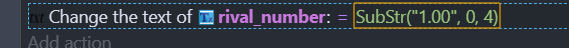# [Solved] There is a decimal number, for example 0.86536636354. I want this number to appear only 2 digits after the comma

there is a decimal number, for example 0.86536636354. I want this number to appear only 2 digits after the comma
and how can i round this decimal number to the nearest integer?

Hiya, for appearing only 2 digits after the comma, see this post:

For rounding off number just use `round()`.
Example: round(4.20) should give you a result of 4, or round (Variable(someNumber)) if you want to round off a variable.

It would be nice if you Search the forum first next time, incase it’s already answered.

1 Like

Try something like this maybe it worksWhere 0 is the start position of your number and 4 is the number of occurrences to display

Gosh, here’s a thread that pretty much covers this question, and it’s been answered in the last 24 hours too! As @reina wrote, you should search the forum first.

1 Like